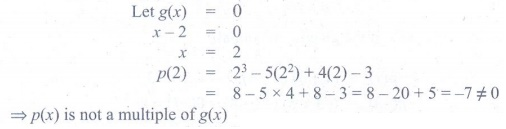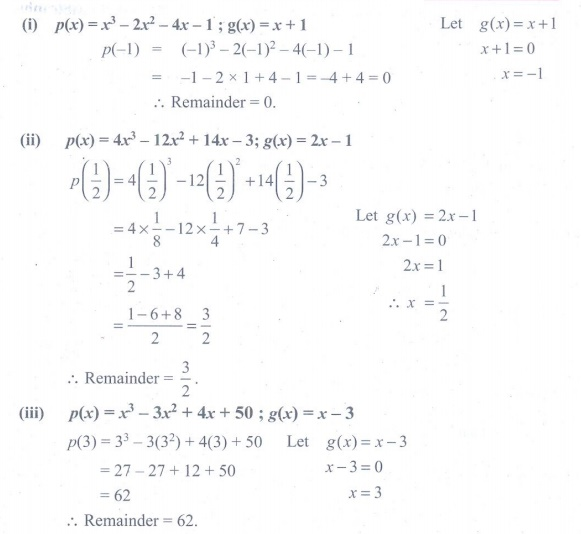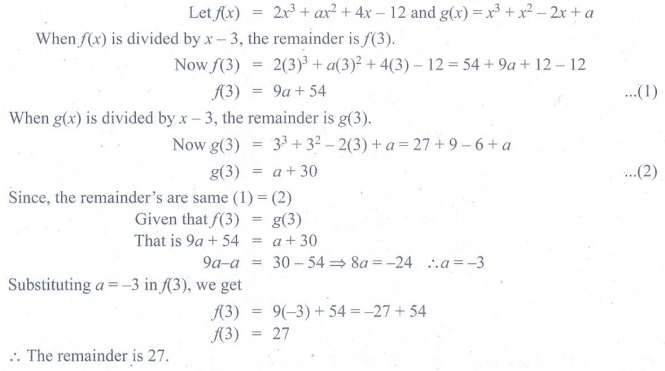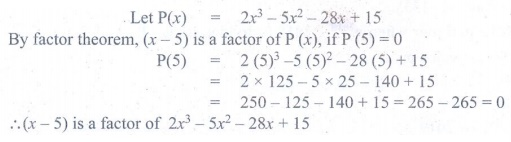Home | | Maths 9th std | Exercise 3.3: Remainder Theorem

# Exercise 3.3: Remainder Theorem

Maths : Algebra: Book Back, Exercise, Example Numerical Question with Answers, Solution : Exercise 3.3: Remainder Theorem

Exercise 3.3: Remainder Theorem

1. Check whether p(x) is a multiple of g(x) or not .

p(x) = x35x2 + 4x − 3 ; g(x) = x – 22. By remainder theorem, find the remainder when, p(x) is divided by g(x) where,

(i) p(x) = x32x24x − 1 ; g( x ) = x + 1

(ii) p(x) = 4x312x2 + 14 x − 3; g( x ) = 2 x − 1

(iii) p(x) = x33x2 + 4x + 50 ; g( x ) = x − 33. Find the remainder when 3x3 − 4x2 + 7x − 5 is divided by (x+3)4. What is the remainder when x2018 +2018 is divided by x–15. For what value of k is the polynomial

p ( x) = 2x3 − kx2 + 3x + 10 exactly divisible by (x–2)6. If two polynomials 2x3 + ax2 + 4x – 12 and x3 + x2 –2x+ a leave the same remainder when divided by (x – 3), find the value of a and also find the remainder.7. Determine whether (x -1) is a factor of the following polynomials:

i) x 3 + 5x 2 – 10x + 4 ii) x 4 + 5x 2 – 5x +18. Using factor theorem, show that (x − 5) is a factor of the polynomial 2x 3 5x2 28x + 159. Determine the value of m , if (x + 3) is a factor of x3 3x2 mx + 24 .10. If both (x -2) and (x – 1/2) are the factors of ax2 + 5x +b , then show that a = b.11. If (x -1) divides the polynomial kx 3 2x2 + 25x 26 without remainder, then find the value of k .12. Check if (x + 2) and (x − 4) are the sides of a rectangle whose area is x2 – 2x – 8 by using factor theorem.Tags : Numerical Problems with Answers, Solution | Algebra | Maths , 9th Maths : UNIT 3 : Algebra
Study Material, Lecturing Notes, Assignment, Reference, Wiki description explanation, brief detail
9th Maths : UNIT 3 : Algebra : Exercise 3.3: Remainder Theorem | Numerical Problems with Answers, Solution | Algebra | Maths